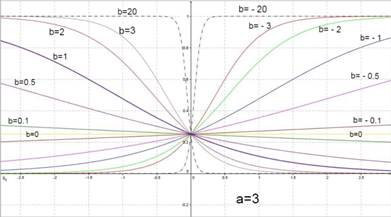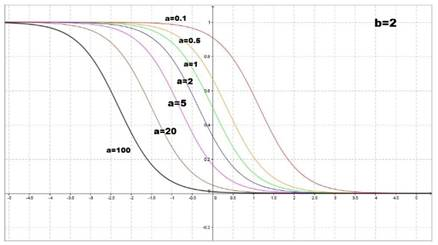# To graph: The members of the family of the function f ( x ) = 1 1 + a e b x , determine the effect of change of b on the graph and affect of a on the graph.### Single Variable Calculus: Concepts...

4th Edition
James Stewart
Publisher: Cengage Learning
ISBN: 9781337687805### Single Variable Calculus: Concepts...

4th Edition
James Stewart
Publisher: Cengage Learning
ISBN: 9781337687805

#### Solutions

Chapter 1.5, Problem 36E
To determine

## To graph:The members of the family of the function f(x)=11+aebx , determine the effect of change of b on the graph and affect of a on the graph.

Expert Solution

### Explanation of Solution

It is given that a>0 . Assume a=3 and graph f(x) for different values of b=20 , b=3 , b=2 , b=1 , b=0.5 , b=0.1 , b=0 , b=0.1 , b=0.5 , b=1 , b=2 , b=3 and b=20 .

The graph is plotted below.Figure (1)

For the given function a is fixed and b is changed.

All the curves take positive values for x .

When b is positive f(x) is decreasing, when b is negative the function is increasing.

When b=0 , f(x) is a constant value for anyx .

Now b is fixed and a is changed.

When b=2 and a=0.5,1,2,3,5,20Figure (2)

The larger the a , the function grows faster when x .

The larger the a , the function decreases slower when x+

And,

When b=2 and a=0.5,1,2,3,5,20Figure (3)

The larger the a , the function grows faster when x+ .

The larger the a , the function decreases slower when x

### Have a homework question?

Subscribe to bartleby learn! Ask subject matter experts 30 homework questions each month. Plus, you’ll have access to millions of step-by-step textbook answers!